Courses

# Test: Solid Mechanics- 3

## 10 Questions MCQ Test GATE Civil Engineering (CE) 2022 Mock Test Series | Test: Solid Mechanics- 3

Description
This mock test of Test: Solid Mechanics- 3 for Civil Engineering (CE) helps you for every Civil Engineering (CE) entrance exam. This contains 10 Multiple Choice Questions for Civil Engineering (CE) Test: Solid Mechanics- 3 (mcq) to study with solutions a complete question bank. The solved questions answers in this Test: Solid Mechanics- 3 quiz give you a good mix of easy questions and tough questions. Civil Engineering (CE) students definitely take this Test: Solid Mechanics- 3 exercise for a better result in the exam. You can find other Test: Solid Mechanics- 3 extra questions, long questions & short questions for Civil Engineering (CE) on EduRev as well by searching above.
QUESTION: 1

### For state, a stress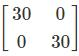mohr circle will have center and radius is

Solution:

σx = 30 : σy = 30 : τxy = 0,

Mohr Circle will be a point with centre (30,0) and radius 0 units

τmax = 0 , radius = 0

QUESTION: 2

### Which of the following is the most appropriate theory of failure for mild steel?

Solution:

Maximum principal stress theory (Rankine’s theory)

According to this theory, permanent set takes place under a state of complex stress, when the value of maximum principal stress is equal to that of yield point stress as found in a simple tensile test.

For design criterion, the maximum principal stress (σ1) must not exceed the working stress ‘σy’ for the material.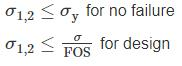Note: For no shear failure τ ≤ 0.57 σy

Graphical representation

For brittle material, which do not fail by yielding but fail by brittle fracture, this theory gives satisfactory result.

The graph is always square even for different values of σ1 and σ2.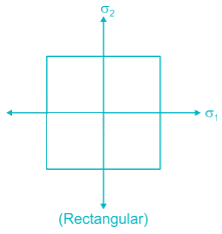Maximum principal strain theory (ST. Venant’s theory)

According to this theory, a ductile material begins to yield when the maximum principal strain reaches the strain at which yielding occurs in simple tension.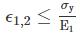For no failure in uni – axial loading.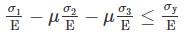For no failure in tri – axial loading.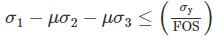For design, Here, ϵ = Principal strain

σ1, σ2 and σ3 = Principal stresses

Graphical Representation

This story over estimate the elastic strength of ductile material.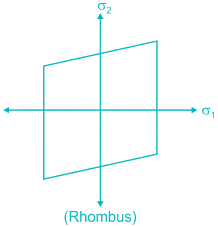Maximum shear stress theory

(Guest & Tresca’s Theory)

According to this theory, failure of specimen subjected to any combination of load when the maximum shearing stress at any point reaches the failure value equal to that developed at the yielding in an axial tensile or compressive test of the same material.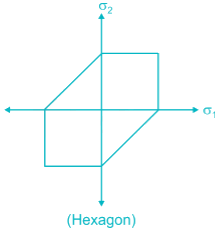Graphical Representation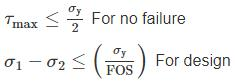σ1 and σ2 are maximum and minimum principal stress respectively.

Here, τmax = Maximum shear stress

σy = permissible stress

This theory gives satisfactory result for ductile material.

Maximum strain energy theory (Haigh’s theory)

According to this theory, a body complex stress fails when the total strain energy at elastic limit in simple tension.

Graphical Representation.This theory does not apply to brittle material for which elastic limit stress in tension and in compression are quite different.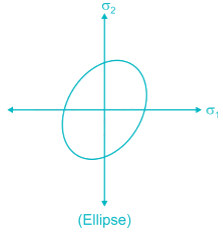Maximum shear strain energy / Distortion energy theory / Mises – Henky theory.

It states that inelastic action at any point in body, under any combination of stress begging, when the strain energy of distortion per unit volume absorbed at the point is equal to the strain energy of distortion absorbed per unit volume at any point in a bar stressed to the elastic limit under the state of uniaxial stress as occurs in a simple tension / compression test.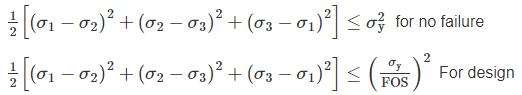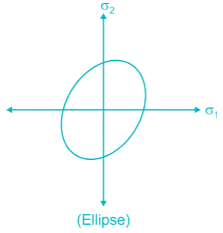It gives very good result in ductile material.

It cannot be applied for material under hydrostatic pressure.

QUESTION: 3

### Consider the following statements: 1. On planes having maximum and minimum principal stress, there will be no tangential stress. 2. Shear stresses on mutually perpendicular planes are numerically equal. 3. Maximum shear stress is numerically equal to half the sum of the maximum and minimum principal stress Which of the following statement is/are correct –

Solution:

On plane of principal stress, shear stress is always zero but in the plane of maximum shear stress, normal stress may exists.

Shear stresses on mutually perpendicular planes are equal due to moment equilibrium.

Maximum shear stress is numerically half of the difference between maximum and minimum principal stress.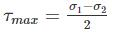QUESTION: 4

A cube is subjected to equal tensile stress on the three faces. If the yield stress of the material is ‘σy’ and poison ratio ‘μ’ then based on strain energy theory, the maximum tensile stress will be

Solution:

Let the maximum tensile stress is σ.

According to maximum strain energy theory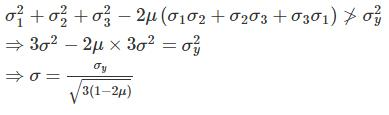QUESTION: 5

At a point in a steel member, major principal stress is 1000 kg/cm2, and minor principal stress is compressive. If uniaxial tensile yield stress is 1500 kg/cm2, then the magnitude of minor principal stress at which yielding will commence, according to maximum shearing stress theory is

Solution:

But σ2 is compressive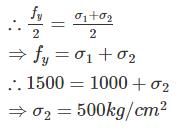QUESTION: 6

The principal stresses developed at a point are +30, -30, 0 MPa. Using shear strain energy theory, a factor of safety obtained is √6. What is yield stress of the material?

Solution:

As per maximum shear strain energy theory,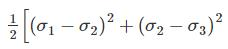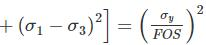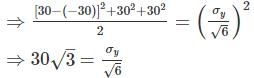σy = 90√2 MPa

QUESTION: 7

In a structural member, there are perpendicular tensile stresses of 100 N/mm2. What is the equivalent stress in simple tension, according to the maximum principal strain theory? (Poisson’s ratio = 0. 50)

Solution:

Equivalent stress

= σ1−μσ2

= 100 – 0.50 × 100 = 50 N/mm2

*Answer can only contain numeric values
QUESTION: 8

In metal forming operation when the material has just started yielding, the principal stresses are σ1 = +180 MPa, σ2 = -100 MPa, σ3 = 0. Following the von Mises criterion, the yield stress is ________ MPa.

Solution:

According to von Mises theory, for yielding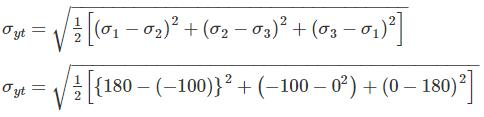σyt = 245.76 MPa

QUESTION: 9

The Mohr’s circle given below corresponds to which one of the following stress condition.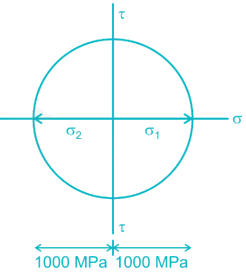Solution:

Radius of Mohr’s circle = 100 MPa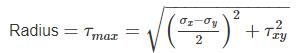And centre of Mohr’s circle is at a distance,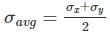from the origin. Here it is in origin.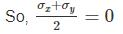As, σ+ σ= 0 both the normal stress may be zero which is a pure shear case or opposite in nature which don’t exist in any of the options. So it is the pure shear case, where the radius is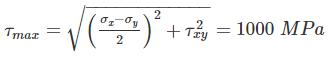*Answer can only contain numeric values
QUESTION: 10

2D stress matrix at a point is given below: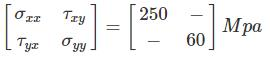If the maximum shear stress is 200 Mpa. The value of τxy will be ________ Mpa.

Solution:

Maximum and Minimum Normal stress is given by: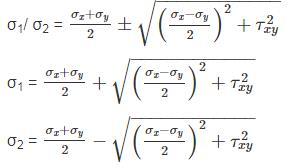σx = 250

σy = 60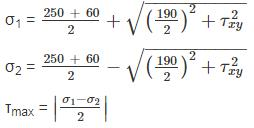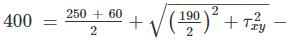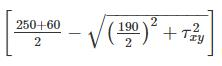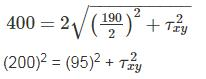τxy = 175.99 Mpa